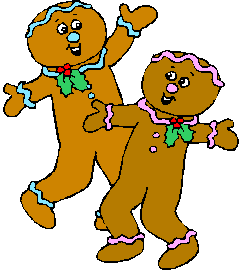#Gingerbread Man Themed Math Pages

Always remember that grade levels are not absolutes -- especially since we're all living in different countries and therefore have different curriculums.  Some kindergarten children will find the Grade One worksheets useful as will some Grade Two students.  Use your judgment!

NOTE:  Magic Squares definition:  add across and down to see the relationships in addition and subtraction.  Great self-checking review (the sums of the answers across = the sums of the answers down making it possible for the children to check their own answers)

1. Two numbers from 0 to 10
2. Fill in the blanks - two numbers from 0 to 10
3. Add a column of three numbers (the first number is always "1")
4. One number from 10 to 19 and one number from 0 to 9   (addition)   (subtraction)
5. Single digit number MAGIC SQUARES  (addition)   (subtraction)
6. Single digit number MATH TABLES  (addition)   (subtraction
7. Single digit number sentences - fill in the + or - sign (addition/subtraction

1. One number from 10 to 99 and one number from 0 to 9   (addition)   (subtraction)
2. Number sentences - fill in the + or - sign (addition/subtraction
3. Two digit numbers - NO CARRYING   (addition)   (subtraction
4. Two digit numbers - CARRYING
5. Fill in the blanks - two digit numbers with CARRYING
6. Two digit number MAGIC SQUARES   (addition)   (subtraction
7. Two digit number MATH TABLES  (addition)   (subtraction
8. Two digit number sentences - fill in the + or - sign (addition/subtraction
9. Add a column of three single digit numbers
10. Two numbers from 0 to 10   (multiplication)
11. Multiplication MATH TABLES  (multiplication)
12. Number sentences - fill in the + or - or x sign (addition/subtraction/multiplication

## DIRECTIONS:

Click one of the links above.  Click once on the image and then print.  To generate a new worksheet, click the GENERATE NEW WORKSHEET link at the top right hand side of your page.